## Concepts and Applications of Multiple regression

By: Ram on Jun 18, 2020

When do you use Multiple Regression?

Based on the scale of measurement, variables can be defined as Binary, Ordinal, Nominal, and Continuous (Ratio and Interval Scale) type. When a decision (or target/dependent) variable is continuous, one of the Statistical Methods available for building the model is multiple regression.  These types of scenarios or problems are classified as Regression problems.

Some of the scenarios and ideas are list below. These examples are across functional areas and business verticals.

Multiple Regression Applications across Industries.

Overall Approach of Regression Model Development

• Objective: What is the objective of a regression model? For example, building a regression model to estimate claim amount given a list of independent variables
• Data Preparation
• In the claim regression model, the data sample can be shared on request.
• Otherwise, you had to think about data sources and variables you will use
• Some of the dimensions of data for a claim scenario are
• Policy Details - when is a policy taken? Insurance amount etc, How many times renewed ..
• Policy Holder Details- Age, gender and others
• Claim Details- how many times claimed in the last 3,6, 9 and 12 months? Summary statistics (min, max, avg, total amount) from claim amount in the last 3,6, 9 and 12 months
• Variable Creation/transformation
• Create dummy variables based on categorical/character variables
• Mathematical Transformation variables - log/sqrt/sqr variables for numeric variables.
• Exploratory Data Analysis (EDA)
• Univariate Analysis (One variable at a time)
• Summary statistics
• Missing Value
• Outliers
• Bivariate Analysis - Claim Amount and Other variables
• Summary claim amount by categorical variable values e.g. average claim amount for Male & Female
• Correlation Analysis - correlation analysis e.g. how the claim amount and age are related
• Model development and validation samples
• 70% development or training sample and 30% validation or testing sample
• Model Development
• Run Multiple Regression
• Select variables which are significant
• Step-wise variable selection
• Assumptions - Residual Analysis and Multi-Collinearity Checks
• Final model - all variable significant (p value less than 0.001), high R2 and adj R2 , over model F statistics & p value significant (p value less than 0.001)
• Score both Development and Validation samples
• Using Model developed, score both development and validation samples
• Check MAPE (Mean Absolute % Error) for Development and Validation samples

Multiple Regression Algorithm: Concepts

A Multiple Regression Problem formulations is of the following form

Y = B0 + B1* X1 + B2 * X2 + B3 * X3…. + Error

Y is Target or Dependent Variable

X1, X2, and X3 etc are set of independent variables or features

B0 is an intercept and B1, B2 and B3 are coefficients for each of the independent variables.

The main aim of the model is to find the values of these parameter estimates.  The method used for estimating parameter values is Ordinary Least Square (OLS).  The method aims to find the values of these parameters such as that the overall error of the model is minimized.

One of the simplest examples of Multiple Regression is Simple Linear Regression in which only one independent Variable is considered and the form will be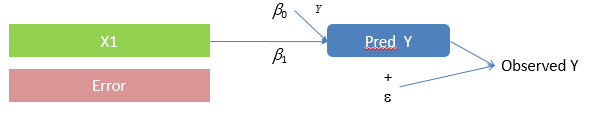Now, we explaining the detailed steps to find values of intercept (B0) and B1, parameter coefficient for X1 variable.

Parameter Estimation in Simple Regression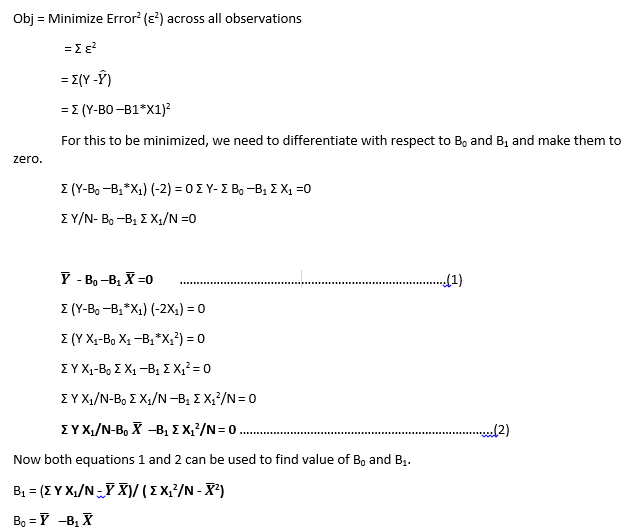Review Multiple Regression Output

Most of the analytical tools (such as Python, SAS, R, and SPSS) gives similar output for a regression model.

A regression model output typically will have 3 parts in the output.

• Analysis of Variance (ANOVA)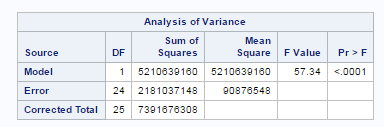• Regression Model Performance Statistics – R2 and Adj R2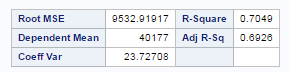One of the key performance statistics for a regression model is R2 indicating % of variance explained by the model. But R2 only increases if you are adding more variables, so Adj R2 is evaluated to not select the complicated model.

• Variable Significance or Parameter Estimates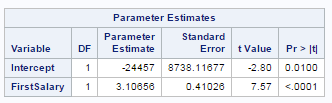The main objective of modeling is to find parameter estimates. Based on T Statistics and P-Value, the variable significance is evaluated. P-Value indicates evidence in favor of the null hypothesis. In the regression model, the null hypothesis is "Beta Coefficient or Parameter Estimate for a variable is Zero". So the lower P-value indicates, the variable can be kept in the model.

Model Selection

• Variable Significance: All variables in the model have to be significant based on the agreed significance level. In practical scenarios, we keep variables that have less than 0.001 P-value but this is a judgment, in other scenarios you may be Ok in selecting variables with P values less than 0.01.
• Variance Explained – R2 and Adj R2 Higher is the better is a model
• Review Multiple Regression Assumptions
• Multi-collinearity: Use VIF can be used to check for multi-collinearity
• Outliers, Independence of Error and Homoscedasticity: Residual Analysis

Tags
Most PopularJun 18, 2020Jul 23, 2020Jun 19, 2020Jun 19, 2021